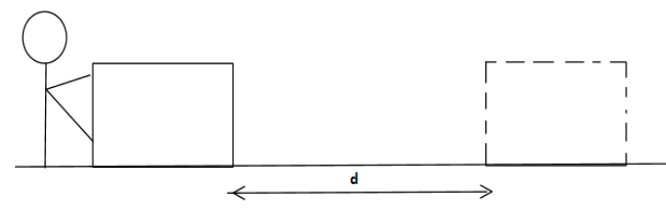Courses
Courses for Kids
Free study material
Free LIVE classes
MoreLIVE
Join Vedantu’s FREE Mastercalss

# Is one joule per second equivalent to one watt ?Verified
259.5k+ views
Hint: In order to answer this question, we must know the basic definition of work done (whose unit is Joule) and power (whose unit is watt) and their SI units, from which we can easily verify the relation given in our question.

Formula used:
$W=F\cdot d$
Where, $W$ is work done, $F$ is force applied and $d$ is distance cover by applying force.
Unit of work is joule.
$P=\dfrac{W}{t}$
where $P$ is power and $t$ is time. Where the unit of power is watt.Now in order to understand this, lets first recap the unit of energy and power,
Watt = SI unit of Power.
Joule = SI unit of Energy.
We know according to mechanics work is nothing but the dot product of force and displacement of a particle or any object.
$W=F\cdot d$ ………… $(1)$
Now if we understand it with the above given figure, consider a man pushing a block by applying a force which transfers energy from him to that block. This energy helps in doing some work by which the given block move from one place to another as shown in figure the block covers distance “d” ,

So from these we can say energy supplied by work done on a block to cover a distance “d” can be in any form like it can be in potential energy or kinetic energy etc.So from above discussion we can say work done is nothing but a form of energy and it can be measured in “Joules”.Hence from above discussion SI unit of work done is “Joule”.

Now we know Power is nothing but the rate of work done or energy supplied to the system.
So according to the above given figure we can say that, On applying energy or doing some work, the distance covered by the object in one second gives power.
Hence, Power is the energy transferred per unit time.
$P=\dfrac{W}{t}$ …………. $\left( 2 \right)$, where “P” is nothing but power and “t” is time.
So from here we can conclude that,
$\text{1watt=}\dfrac{\text{1joule}}{\text{1second}}$ …………… $\left( 3 \right)$
So, from equation $\left( 3 \right)$ we can say one joule per second is equivalent to one watt.

Note: In this question to be more clear we can say, Energy decides how far the block can be pushed, while power decides how much faster the block can be pushed, the example of block is just for understanding purposes we can take any other scenario also. SI unit “joule” is given in the name of English physicist Prescott Joule, while “watt” as SI unit is accepted in the name of Scottish chemist James Watt.
Last updated date: 01st Oct 2023
Total views: 259.5k
Views today: 4.59k